### Home > PC > Chapter 2 > Lesson 2.3.4 > Problem2-113

2-113.
1. At noon, John is 60 miles directly south of Cactus Junction and driving north at 40 miles per hour. At the same time, Amy is 20 miles west of Cactus Junction and driving east at 30 miles per hour. Homework Help ✎

1. Express the distance of John's car north of Cactus Junction as a function of time.

2. Express the distance of Amy's car east of Cactus Junction as a function of time.

3. Find the distance between John and Amy at the time t hours after noon.

4. When are John and Amy's cars closest together? (Hint: Graph the distance equation you found in part (c).)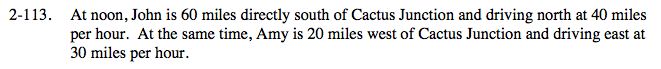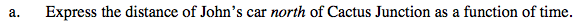How far away is John from North now?
What expression describes his distance?

−60 + 40t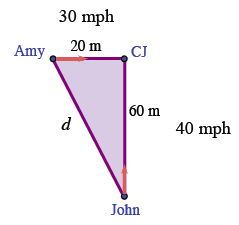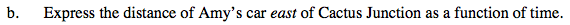Follow the example for John's distance.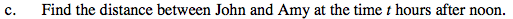Use the distance formula.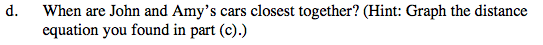t = 1.2 hours or at 1:12 p.m; The distance between them is 20 miles.